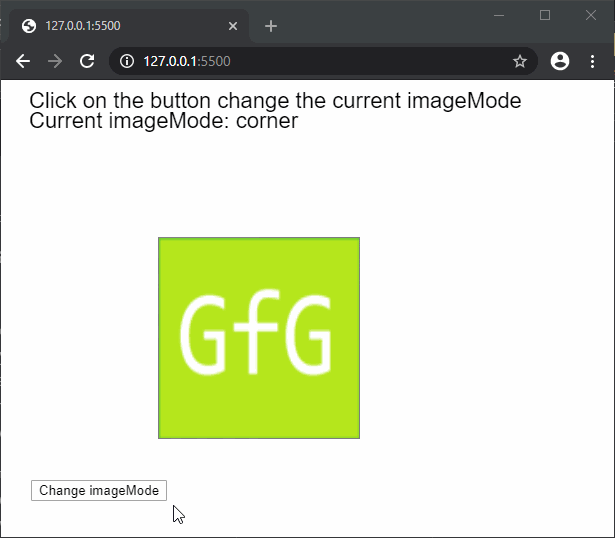GFG App
Open AppBrowser
Continue

The imageMode() function is used to set the image mode of an image. The image mode defines the position of the image in the canvas, by changing the way that the parameters given to the image() function are interpreted.

Syntax:

`imageMode( mode )`

Parameters: This function accepts a single parameter mode that defines the mode to be used. It can have the following values:

• CORNER: This mode interprets the second and third parameters given to image() as the upper-left corner of the image.
• CORNERS: This mode interprets the second and third parameters given to image() as the upper-left corner and the fourth and fifth parameters as the bottom-right corner of the image.
• CENTER: This mode interprets the second and third parameters given to image() as the center point of the image. Additonally, if the fourth and fifth parameters are specified, they are used as the width and height of the image.

Below example illustrate the imageMode() function in p5.js:

Example:

 `function` `preload() { ` `  ``img = loadImage(``'sample-image.png'``); ` `} ` ` `  `function` `setup() { ` `  ``imageModes = [ ` `    ``CORNER, ` `    ``CORNERS, ` `    ``CENTER ` `  ``]; ` `  ``i = 0; ` `  ``currMode = imageModes[i]; ` ` `  `  ``createCanvas(600, 400); ` `  ``textSize(22); ` ` `  `  ``// Create a button for the switching ` `  ``// the imageMode ` `  ``switchBtn = createButton(``"Change imageMode"``); ` `  ``switchBtn.position(30, 400) ` `  ``switchBtn.mousePressed(switchMode); ` `} ` ` `  `function` `draw() { ` `  ``clear(); ` `  ``text(``"Click on the button change the current"``+ ` `                           ``" imageMode"``, 20, 20); ` `  ``text(``"Current imageMode: "` `+ currMode, 20, 40); ` ` `  `  ``// Creating a rectangle to demonstrate ` `  ``// the location of the image ` `  ``rect(150, 150, 200, 200); ` ` `  `  ``// Setting the imageMode ` `  ``imageMode(currMode); ` ` `  `  ``// Drawing the image ` `  ``image(img, 150, 150, 200, 200); ` `} ` ` `  `function` `switchMode() { ` `  ``// Change the current imageMode ` `  ``if` `(i < imageModes.length - 1) ` `    ``i++; ` `  ``else` `    ``i = 0; ` `  ``currMode = imageModes[i]; ` `} `

Output:Online editor: https://editor.p5js.org/

My Personal Notes arrow_drop_up